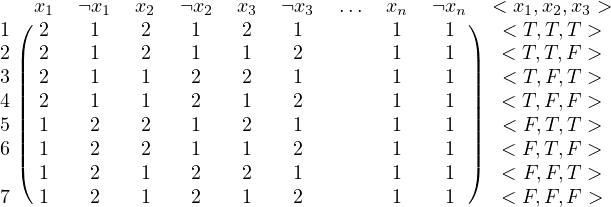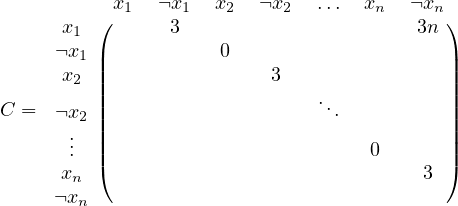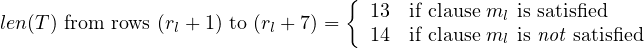# The Column Problem

You’re at the terminal, paging through a csv file, with a vague idea of what you’re looking for. It’s a mess: the data is all commas and quotes, without any whitespace to line columns up. You google, and pass the csv to `column -t` to pretty-print the csv as a table. And it’s still a mess. No combination of options readably displays the table on your terminal’s 80 columns.

The way to lay out the table seems obvious: choose the column widths that maximize avilable space by minimizing the length of the table.

Motivated by such low-hanging fruit, I set out to develop a quick algorithm that would optimize column widths for any table. This would lead me down a different path than expected, as I discovered that this is (SPOILER ALERT) actually an NP-hard problem. If you’d like to follow my problem-solving process, you can keep reading, or you can jump to a proof of NP-hardness at the end.

## Let’s see what it costs

To get an initial handle on the column-width optimization problem (COL-OPT from here on), let’s start by defining a cost function for a table. The cost function will take a table and proposed column widths as input and return how many lines it would take to print the table out.

This could be written like so in Python:

``````# table  is a 2D array of type str[r][c]
# widths is a 1D array of type int[c]
def cost(table, widths):
table_len = 0
for row in table:
row_len = 0
for (column, width) in zip(row, widths):
cell_len = math.ceil(1.0 * len(column) / width)
row_len = max(row_len, cell_len)
table_len += row_len
return table_len
``````

Translating that to English, “Find the length of the table by adding up the length of all the rows. Find the length of a row by looking at all cells in the row, and finding the cell that needs the most lines using the proposed widths.”

For example, given the following arguments:

``````table = [
["abcd", "abcdefgh", "abcdefgh", "abcd"], # 4, 8,  8, 4
["abcd", "abcd", "abcdefghijkl", "abcd"], # 4, 4, 12, 4
["abcdefg", "abcde", "abc", "abc"],       # 7, 5,  3, 3
]

widths = [2, 3, 5, 2] # total width = 12
``````

Calling `cost(table, widths)` returns 10, the sum of the lines filled by the individual rows: `3 + 3 + 4 = 10`.

If you’ll excuse a quick Python aside, this function maps well to a list comprehension, yielding the following one-line definition:

``````# Yes, I know this is bad
def cost_one_liner(table, widths):
return sum([max([math.ceil(1.0 * len(column) / width) for (column, width) in zip(row, widths)]) for row in table])
``````

At first glance, COL-OPT seems simple to optimize. My intuition says that I can find an optimal substructure that will let me break this problem apart for a dynamic programming solution.

If there is an optimal substructure, it will be found by breaking the table apart at column boundaries, optimizing the column widths of sub-tables, and then recombining solutions to the sub-tables for a global solution.

## (Non-)Optimal Substructure

Following this strategy, I spent many sheets of scratch paper looking for a solution, but kept finding exponential solutions instead of polynomial ones.

This made me suspicious. I decided to look at the problem from a brute-force perspective and see if I could use combinatorics to count all possible column splits.

This yields the following observation:

Consider a table with `c` columns that you’d like to fit into a terminal `w` characters wide. In this case, there are `w-1` possible locations that can serve as column dividers. Of those `w-1` locations, only `c-1` will serve as column dividers.

To illustrate, here’s an example assignment when a table with 3 columns is fit into a terminal of width 5:

``````c = 3, w = 5

|  *     *     |    c-1 = 2 column dividers to choose
----------------
|  |  |  |  |  |      w = 5 spaces
----------------
|  ^  ^  ^  ^  |    w-1 = 4 locations for colum dividers
``````

This yields the binomial expression `w-1 choose c-1`, which is Big O exponential in terms of `w`, depending on the value of `c`.

Keeping this result in mind, I was drawn back to the cost function, wondering what about it prevented me from optimizing sub-tables and recombining them later.

This path led me to zero in on the cost function for an individual row.

Looking at the relevant lines from the cost function above:

``````        for (column, width) in zip(row, widths):
cell_len = math.ceil(1.0 * len(column) / width)
row_len = max(row_len, cell_len)
``````

For an individual row, its cost is a maximum value computed by looking at all columns in the row.

Understanding that there is an exponential number of column splits to consider, and that computing the cost for each row necessarily needs to include all columns, was a lightbulb moment for me to see this as a fundamentally exponential problem.

Once I made this connection, I gave up on finding an optimal substructure. This also changed the goal of the problem. Instead of finding a polynomial solution with dynamic programming, I’d like to prove that no polynomial solution exists.

## Prove it

One way to show that a polynomial solution (probably) can’t be found is to prove that this problem belongs to the NP-hard family.

To show this, I would need to build a reduction from an NP-hard problem to COL-OPT, demonstrating that a solution to an NP-hard problem can be translated into an instance of COL-OPT in polynomial time.

So, I set out to prove that COL-OPT is NP-hard, working with the NP-hard problem that I find easiest to wrap my head around, satisfiability. In a variant of satisfiability called “3-conjunctive normal form satisfiability” (or 3-CNF-SAT) by CLRS, a boolean formula consisting of clauses with three variables each is given as input. A solution is a truth assignment to the boolean variables that satisfies all clauses in the formula.

Trial-and-error produced many almost-there solutions. I felt confident that the table built for COL-OPT would need columns for each variable and it’s negation. However, all my attempts suffered from the same problem: I could find no way of enforcing a valid truth assignment, where the construction of the table guarantees that no variable will be assigned the same value as its negation—a paradox.

## Re-Parameterize

To enforce this constraint, I chose to parameterize COL-OPT with a constraint matrix `C` instead of the previous integer `w` that simply gave the width the table could fill. This matrix generalizes on the plain width, in that it’s simple to define the original problem, while allowing for additional constraints on the widths of subsequences of columns.

It felt like cheating when I initially considered changing the paramters to the problem. However, since the updated constraint matrix allows for a simple stating of the original problem, and reduces the complexity of the problem by reducing the number of column splits to be considered, I considered it to be an acceptable change.

With this new parameter, the rest of the solution fell into place, giving the proof below. So, a fairly circuitous path, but I arrived at a solution that I’m happy with!

## The Proof

[Note: this was generated from LaTeX source, a pdf is also available]

Definitions

An instance of the Column-Width Optimization (COL-OPT) problem consists of the following:

• A row by column (r×c) matrix T, where each cell in T is empty or holds a sequence of characters
• A c × c constraint matrix C, where each cell is C holds a non-negative integer.

A solution to COL-OPT is given by the c-length vector w, where w:

• Gives the widths for each column in T such that the length of table T is minimal. The length of T is given by the sum of the length of each row. The length of a row is given by the maximum value of a cell divided by its assigned column width for all cells in the row.

len(T) = i=1r maxj=1clen(T[i][j])w[j]

• Assigns each column a width of at least 1.

for 1 i c, w[i] > 0.

• Satisfies constraint matrix C so that if C[i][j] is non-zero, the sum of all column weights from i to j is equal to C[i][j].

for 1 i c,i j c, if C[i][j] is non-zero, k=ijw[k] = C[i][j].

From this definition of w, constraint matrix C must give a valid total width for the table. C[c] c.

Define the “3-conjunctive normal form satisfiability” problem (3-CNF-SAT) as defined in CLRS [Introduction to Algorithms, 3rd ed., p. 1082].

3-CNF-SAT defines:

• A literal in a boolean formula is an occurrence of a variable or its negation.
• A boolean formula is in conjunctive normal form, or CNF, if it is expressed as an AND of clauses, each of which is the OR of one or more literals.
• A boolean formula is in 3-conjunctive normal form, or 3-CNF, if each clause has exactly three distinct literals.

An instance of 3-CNF-SAT consists of a boolean formula ϕ.

Theorem

Optimization of column widths for a table given by COL-OPT is NP-hard.

Proof

Reduction from 3-CNF-SAT to COL-OPT

Prove that COL-OPT is NP-hard by reducing 3-CNF-SAT to it.

Let τ(ϕ) be a reduction from 3-CNF-SAT to COL-OPT.

Given m clauses of n unique variables in ϕ, construct a 7m×2n table T. Interpret the 2n columns as representing each possible literal. Construct the rows of the table in multiples of seven for each clause ml in ϕ, where each clause ml consists of three boolean variables.

With three boolean variables, there are 23 = 8 possible truth assignments. Of the eight possible assignments, all but one will satisfy ml. Construct a row for each of the seven truth assignments which satisfy ml, where a cell in the table with length 2 represents a truth assignment and length 1 a false assignment. All variables not represented in clause ml have length 1.

For example, given the clause (x1 x2 ∨¬x3), construct the following 7 rows from the corresponding truth assignments:Note that the row which is excluded is the row which corresponds to the assignment < x1,x2,x3 > = < F,F,T >, since this is the only assignment which fails to satisfy the clause.

Construct the constraint matrix C starting with a 2n × 2n zero matrix. Starting with i = 1, for i < 2n and increasing i in increments of 2, let C[i][i+1] = 3. Let C[2n] = 3n.

This produces the matrix:Together, the matrices T and C give an instance of the COL-OPT problem. This describes the reduction τ in full. Furthermore, it is evident that τ(ϕ) is polynomial, since m and n bound the size of ϕ and producing the 7m × 2n table T is polynomial in terms of m and n.

Proof of Equivalence

To prove that τ is a valid reduction, let T and C be an instance of COL-OPT produced by τ(ϕ), where ϕ is an instance of 3-CNF-SAT. Let w be the vector of column weights subject to C that minimizes the length of T.

Following the construction of T, w consists of n pairs of weights. Subject to C, for each pair of weights there are two possible assignments: < 2,1 > or < 1,2 >. This forces each column pair, representing a variable and its negation, to be a valid truth assignment.

Consider the cost function len(T) that w minimizes. Following the construction of T, each sequence of 7 rows corresponds to a clause ml in ϕ, and will have cells with length 2 restricted to exactly 3 column pairs.

Since every column must have a width of at least 1, cells with length 1 have no effect on the cost function for a row. Therefore, for every 7 rows we can compute the cost entirely in terms of the 3 column pairs represented by the 3 variables in ml.

Suppose the truth assignment given by w satisfies the clause. Following the construction of T, this assignment must be represented as one of the rows in the sequence. The row which matches the truth assignment will have length 1, while all other rows have length 2, giving the cost: 1 + 2 6 = 13.

On the other hand, suppose this truth assignment does not satisfy the clause. In this case, the assignment is not represented in one of the seven rows in T, so all rows have length 2, giving the cost 2 7 = 14.

For 1 l m, let rl = 7(l 1) give the row index for each sequence of 7 rows. For all rl,Note that computing the cost for each clause’s rows is independent of all other clauses.

Therefore, if w minimizes the length of table T globally, it also minimizes the number of clauses which are dissatisfied. Conversely, it maximizes the amount of satisfied clauses.

This gives the result that if ϕ is satisfiable, the length of T will be exactly 13m, since every m sequence of 7 rows must have length 13. If the length of T > 13m, ϕ is not satisfiable, since at least one clause was not satisfied.

if ϕ 3-CNF-SAT, τ(ϕ) COL-OPT.

Conversely, suppose τ(ϕ) COL-OPT.

Following the definition of τ and COL-OPT, the weights w which minimize T will give a length such that 13m len(T) 14m.

If len(T) = 13m, then each sequence of 7 rows must have length 13. Given each sequence of 7 rows, the clause that would produce this sequence can be determined following the construction in τ. Furthermore this clause will be satisfied with the truth assignment given by w.

if τ(ϕ) COL-OPT, ϕ 3-CNF-SAT.

Therefore, since a polynomial reduction from 3-CNF-SAT to COL-OPT exists, COL-OPT is NP-hard.

## Closing Thoughts

With the proof that COL-OPT is NP-hard, there’s a few more directions worth exploring:

• This problem went in an unexpected direction, in that I now have a nice proof instead of a working program. Understanding the limits of the problem, what’s the best exponential solution I can come up with?

• A proof of NP-hardness is a fairly large hammer to wield. Consider proving this problem has non-optimal substructure, which may be simpler than the NP-hard proof while still demonstrating that dynamic programming is not the solution of choice.

• I’m irked that I couldn’t find a proof with the original paramterization of the problem: a plain integer `w` instead of the constraint matrix `C`. Can I find a proof using the original parameterization?